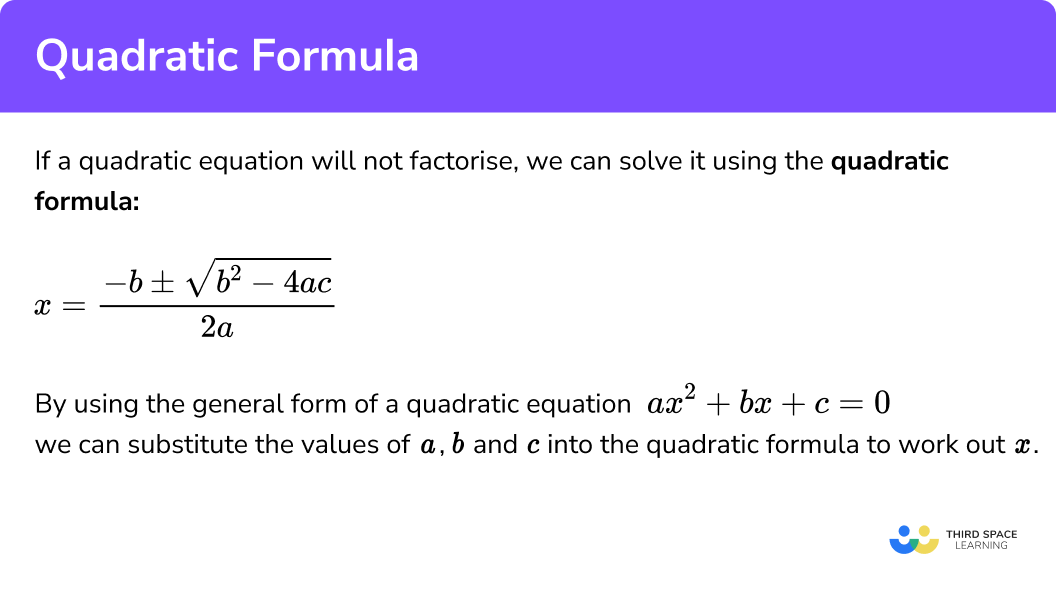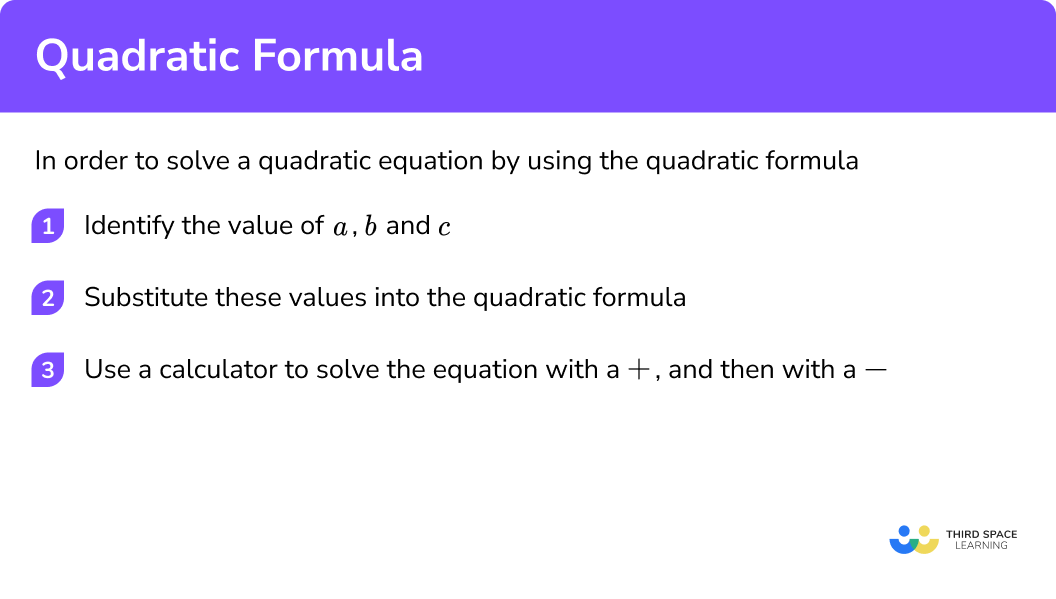Here we will learn about the quadratic formula and how we can use it to solve quadratic equations.

There are also solving quadratic equations worksheets based on Edexcel, AQA and OCR exam questions, along with further guidance on where to go next if you’re still stuck.

## What is the quadratic formula?

The quadratic formula is a formula that provides the solutions to quadratic equations.

$x=\frac{-b\pm\sqrt{b^2-4ac}}{2a}$

By using the general form of a quadratic equation:

$a x^{2}+b x+c=0$

we can substitute the values of a, b and c into the quadratic formula to work out x.

We need to memorise the quadratic formula.

### What is the quadratic formula?#### The ± in front of the square root means ‘plus or minus’

Quadratic equations normally have two solutions, so we need to use the formula twice, once with a + and once with a −.

\begin{aligned} x=\frac{-b+\sqrt{b^2-4ac}}{2a}\\ \\ x=\frac{-b-\sqrt{b^2-4ac}}{2a} \end{aligned}

The solutions to quadratic equations could involve fractions, decimals or integers.

### Related lessons on quadratic equations

Quadratic formula is part of our series of lessons to support revision on quadratic equations and solving equations. You may find it helpful to start with the main solving equations lesson for a summary of what to expect, or use the step by step guides below for further detail on individual topics. Other lessons in this series include:

## How to use the quadratic formula

In order to solve a quadratic equation by using the quadratic formula, it is a good idea to simplify it and make sure it is in the form of the general quadratic equation.

$ax^{2}+bx+c=0$

We must ensure the quadratic equation is equal to 0, rearranging it if necessary.

Step by step guide:

1. Identify the value of a, b and c.
2. Substitute these values into the quadratic formula.
3. Use a calculator to solve the equation with a + and then with a −.

Step-by-step guide: Substitution

### Explain how to use the quadratic formula in 3 steps### Example 1: with a = 1

Solve

$x^{2}-8x+15=0$

1. Identify the a, b and c.

$a=1,\qquad b=-8,\qquad c=15$

2Substitute these values into the quadratic formula.

$x=\frac{-b\pm\sqrt{b^2-4ac}}{2a}$

$x=\frac{-(-8)\pm\sqrt{(-8)^2-4(1)(15)}}{2(1)}$

Using brackets will help to make the calculation clear.

3Use a calculator to solve the equation with a +, and then with a −.

\begin{aligned} x&=\frac{-(-8)+\sqrt{(-8)^2-4(1)(15)}}{2(1)}\\ x&=5\\ \\ \\ x&=\frac{-(-8)-\sqrt{(-8)^2-4(1)(15)}}{2(1)}\\ x&=3 \end{aligned}

When we plot the graph of the quadratic equation we get a special ‘U’ shaped curve called a parabola.

By using graphing techniques such as this we can see that the roots of the quadratic equation are where the quadratic graph crosses the x-axis.

We can check that our solution is correct by substituting it back into the quadratic function.

### Example 2: with a > 1

To solve

$2x^{2}+5x+3=0$

$a=2,\qquad b=5,\qquad c=3$

$x=\frac{-b\pm\sqrt{b^2-4ac}}{2a}$

$x=\frac{-(5)\pm\sqrt{(5)^2-4(2)(3)}}{2(2)}$

Using brackets will help to make the calculation clear.

\begin{aligned} x&=\frac{-(5)+\sqrt{(5)^2-4(2)(3)}}{2(2)}\\ x&=-1\\ \\ \\ x&=\frac{-(5)-\sqrt{(5)^2-4(2)(3)}}{2(2)}\\ x&=-1.5 \end{aligned}

We can see the roots of the quadratic equation are where the quadratic graph crosses the x-axis.

We can check that our solution is correct by substituting it into the original equation.

### Common misconceptions

• When to use the quadratic formula

We can use the quadratic formula to solve quadratic equations regardless of whether the factorising (sometimes called factoring) method will work.

• Not including the sign in front of a, b and c

When writing the coefficients of the variables for the values of a, b and c, we need to include the sign in front but not the variable.

$x^{2}-8x+15=0$

So here b = − 8, NOT 8 or 8x

• Typing into calculator

Mistakes can be made when typing into a calculator.

E.g. for

$2x^{2}+5x+3=0$

$a=2,\qquad b=5,\qquad c=3$

Use the fraction button and brackets to carefully type into the calculator.

Use the arrow buttons to change the + on the top left of the calculation to a − to work out the second solution.

• Sometimes there are no real roots to a quadratic equation

E.g.

$x^{2}-4 x+5=0$

We can see that there are no values of x that give a y value of 0.

The graph does not cross the x-axis.

### Not needed at GCSE but very interesting!

There is an easy way to find out how many real roots a quadratic equation has by using the expression under the square root in the quadratic formula:

$x=\frac{-b\pm\sqrt{b^2-4ac}}{2a}$

This is called the discriminant:

$b^{2}-4ac$

• If
$b^{2}-4 a c>0$

this means there are two solutions (these are real numbers).

• If
$b^{2}-4 a c=0$

this means there is one solution (which is a real number).

• If
$b^{2}-4 a c<0$

this means there are no real solutions, here the solutions will be imaginary numbers.

1. Solve:

{x}^2+4x+3=0We can use the quadratic formula with a=1, b=4 and c=3 . Make sure you find both solutions using the formula.2. Solve:

{x}^2+3x-18=0We can use the quadratic formula with a=1, b=3 and c=-18 . Make sure you find both solutions using the formula.3. Solve:

2{x}^2-3x-2=0We can use the quadratic formula with a=2, b=-3 and c=-2 . Make sure you find both solutions using the formula.4. Solve:

3{x}^2-4x-5=0We can use the quadratic formula with a=3, b=-4 and c=-5 . Make sure you find both solutions using the formula.1. Solve:

x^{2}+2x-4=0

(3 marks)

(1)

x=1.24 (2.d.p)

(1)

x=-3.24 (2.d.p)

(1)

2. Solve:

2x^{2}-3x+1=0

(3 marks)

Substitution into the quadratic formula or an attempt to factorise

(1)

x=1

(1)

(1)

3. Solve:

3 x^{2}+6 x-2=0

(3 marks)

(1)

x=0.29 (2.d.p)

(1)

x=-2.29 (2.d.p)

(1)

### Did you know?

• Did you know that Al-Khwarizmi (Abu Ja’far Muhammad ibn Musa al-Khwarizmi) was one of the first people in history to write about algebra? He lived in Baghdad around 780 to 850 AD and his book “Hisab Al-jabr w’al-muqabala” is where we get the word ‘algebra’ (meaning ‘restoration of broken parts’).

• Did you know the ancient Babylonians could solve quadratic equations using a method equivalent to the quadratic formula, despite not using algebraic notation!

The history of mathematics is amazing!